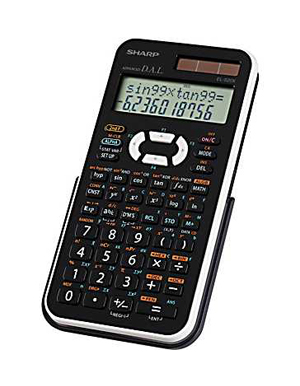Calculator BrandsSharp EL-520XBWH Engineering/Scientific Calculator

Product Features
• Direct Algebraic Logic allows students to enter the elements of an expression in the exact order they appear in the textbook
• Extra large 12-digit, 2-line display - one line equation and one line numeric
• Multi-Line Playback allows students to playback expressions and substitute new numeric values
• Differential and integral calculus functions
• Hard case protects case for long-term durability

Product Description

The EL-520XBWH 12-digit scientific calculator by Sharp performs over 419 advanced scientific functions. It features Multi-Line Playback to make scientific equations easier for students to solve. Once an answer is derived, students can "playback" the expressions and substitute new numeric values. The EL-520XBWH is ideal for students studying general math, algebra, geometry, trigonometry, and calculus. In addition, it can be used for statistics, biology, chemistry, and physics.

Price : \$24.99LINKSDISCLAIMERCONTACT US